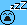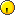# n largest sum##n largest sum

two arrays of length n (decreasingly sorted) .now take an element from the first array and another from the second and add them both ....
the aim is to find such n largest sums in o(n) complexity

##Re: n largest sumLast edited by ankurgutpa on Sun Aug 17, 2008 1:31 pm; edited 1 time in total

##@PAPA

how can it be 2n-1 largest sum
a+b can be smaller than a+b

##@Etawah

1. sum=a+b
2.i=0,j=0,k=0
3. while(--n),
4.{ if(a[i]-a[i+1] > b[j]-b[j+1])
j++;
else
i++;
k++;
sum[k]=a[i]+b[j];
}##@CHACHI

chunky samjhao bhai......

##Re: n largest sum

chunky's solution is wrong
i am unable to see any O(n) solution but optimized it as follows
let A={a,b,c,d}
B={p,q,r,s}
now consider the matrix of sums :
a+p a+q a+r a+s
b+p b+q b+r b+s
c+p c+q c+r c+s
d+p d+q d+r d+s
...
This matrix is sorted in decreasing order row-wise and column-wise
now largest n sums will be found in upper triangular matrix ie from n(n+1)/2 elements and remaining lower half n(n-1)/2 elements can be neglected. Now there can be at most 1 element (nth largest) from non-major diagonal ( ie max (a+s, b+r,c+q,d+p) ) This is O(n) step.Say we find out this element then also it is possible that this element does not exist in n largest sumnow etawah. tell us the answerLast edited by ankurgutpa on Wed Aug 20, 2008 8:29 am; edited 1 time in total

##implementation of that algo

we have to find n largest sums, which we can make using 1-1 elements from both arrays....???
if yes then ...try this,if anything is wrong then let me know:
otherwise explain me the question.

#include<iostream>
using namespace std;
int main()
{
int n,n1,i,j,k;
cin>>n;
n1=n;
int arr1[n],arr2[n],sum[n];
for(i=0;i<n;i++) cin>>arr1[i];
for(i=0;i<n;i++) cin>>arr2[i];
sum=arr1+arr2;
i=0,j=0,k=0;
while(--n)
{
if(arr1[i]-arr1[i+1] > arr2[j]-arr2[j+1])
j++;
else if(arr1[i]-arr1[i+1] == arr2[j]-arr2[j+1])
{
if(arr1[i]>arr2[j])
j++;
else
i++;
k++;
sum[k]=arr1[i]+arr2[j];
}
else
i++;
k++;
sum[k]=arr1[i]+arr2[j];
}
for(i=0;i<n1;i++)
cout<<i+1<<" largest sum:"<<sum[i]<<"\n";
cin>>i;
}

##@All

haan yaar ankur...galat aa rha hai.....
koi decode karo yaar.....Last edited by shivang on Wed Aug 20, 2008 10:29 am; edited 1 time in total

##Re: n largest sum

it's wrong again
chk for n=5
10,8,6,4,2
5,4,3,2,1##Re: n largest sum